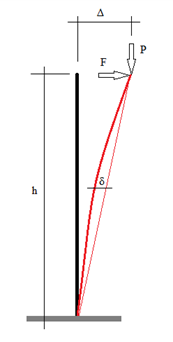# # P-Δ vs. P-𝛿

## # Overview

A P-delta analysis considers the effect of deflection on the loads carried by the structure: the difference between the two options being that the P- effects are global while the P- effects are local.

The features introduced to the design layer in GSA 10.1 make it much more straightforward to consider both P- and P- effects.

## # What are P-Δ and P-𝛿 effects?

To highlight the difference, consider a simple vertical column of height h subject to a transverse force F at the top and a vertical load P there will be a linear bending moment distribution from 0 at the top to at the base.### # Global

In a linear analysis there is no interaction between the axial and transverse load. However, the effect of this transverse load is to create a deflection at the top of the column. If this deflection is then there is an additional moment at the base of the column of , so that the total base moment is:

This is the P- (or P-big-delta) effect that modifies the response of the structure.

Note: If the column is in tension the P- effect reduces the moment.

### # Local

While this takes into account the global effect of the interaction of load and deflection, there is a local effect which this misses. The way the element deflects means that the moment at mid height can be more than that predicted by the P- effect.

At mid height there is an additional moment of . This is the P- (or P-small-delta) effect.

While this is not important for the response of the structure as a whole it can be significant to the design of individual members, especially if this effect coincides with the position of maximum bending in the member.

## # When are P-Δ and P-𝛿 important to consider?

### # P-Δ

Typically P- effects are important when a structure is susceptible to buckling. This is usually the case if the buckling load factors are < 10. In these situations a P- analysis should be selected rather than a linear analysis.

Note: When the analysis is no longer linear, combination cases are not valid. Take care to define the design loads for the analysis cases rather than base load cases (e.g., dead, live, etc.).

### # P-𝛿

P- is significant when displacements are large or columns are slender. The P- effect is important for local buckling and for design algorithms that expect member buckling to be accounted for by analysis.

This includes the AISC direct-analysis method, removing the need for a side sway buckling analysis to determine K.

See Static P delta analysis options for more details on how GSA calculates these forces.

## # How to adjust your GSA model to account for P-Δ and P-𝛿 effects

P- effects can be captured using a Static P delta analysis. If the structure requires large deflection assumptions (if stiffness matrix must be generated from deflected geometry), a nonlinear static analysis is recommended.

P- effects can be captured by subdividing members into multiple elements. If a model is built in the GSA design layer column, members are generally defined from floor to floor. The simplest way to pick up the P- for analysis is to split the columns into two or more elements.

To do this:

1. Select the column members.
2. Set the element size to approximately half (or an appropriate fraction of) the storey height.
3. When using the Create elements from members tool, GSA will generate multiple elements per column allowing the analysis to pick up both the P- and P- effects.

Remember: P- effects are global. P- effects are local.